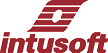STATESET, on the other hand creates a new circuit branch in which the element output is initialized during the entire DC operating point calculation. Once the operating point is calculated, the input of the STATESET branch connects to its output so the AC and Transient analyses can proceed. STATESET is essentially a gain element that has 2 terminals, input and output, where a signal flows from the input to the output in control system fashion. STATESET is a very simple concept, however, standard SPICE simulators can't handle the problem. Users of XSPICE enabled simulators can easily add a "code model" that accomplishes the desired result. Other SPICE simulators (Pspice and Hspice) need to add a new primitive element. One of the most daunting nonlinearities is the "Middlebrook" equation  that describes a peak current controller as a function of average current and its terminal voltages. This equation has more than one stable solution, which can cause the SPICE DC operating point solution to become unstable, and therefore unable to converge. This web page describes the model with example drawings can be downloaded. The new STATESET model ships with our latest update, Build 3684.  Middlebrook, R.D. "Modeling Current-Programmed Buck and Boost Regulators, IEEE Transactions on Power Electronics,vol PE-4, 1989 pp. 36-52.

 A Linear PWM MODEL: A pulse width modulator average model sets output voltage and input current to functions of the input duty ratio. The simplest of all models results when the switched inductor current is continuous, CCM, continuous conduction mode. The process is governed by Z-Transform theory, requiring a zero-order hold and a limiter to constrain the duty ratio to be between 0 and 1. The current and voltage functions are nonlinear; but they can be made linear for the DC solution by using a STATESET element as shown below: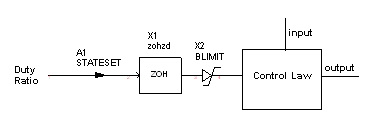Normally, the duty ratio will depend on the output of a controller that senses the output current and voltage. The control law typically depends on the input and output voltage, making the overall solution nonlinear. But the STATESET element uses a constant for the duty ratio during calculation of the DC operating point; which effectively makes the system of equations linear, thus guaranteeing a unique solution. Downloads:      Examples, ICAP/4 Drawings      Library and symbols, ".lib" and ".sym" files      CML.dll (XSPICE plugin)      Literature Inside the Model: The following switch representation is used to make a model that relies on the superposition of the solutions for the 3 switch positions shown.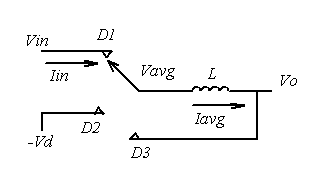For superposition to work, the driving point impedances for each position cannot be affected by the switch position. If that's true, then:(1) and(2) For a complete development of these equations please download the literature. The Buck Model: The buck regulator, shown below, is used To reduce the input voltage. The D1 switch is driven and the D2 switch is constructed using the freewheeling diode. In CCM, D3=0. As the load current is reduced, the regulator drops into DCM and D3 is greater than 0.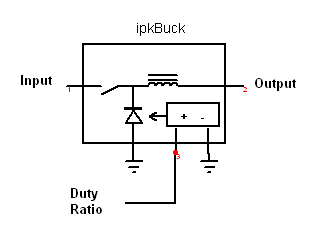The Boost Model: Shown below, this circuit is used to increase the input voltage, reversing Vo and Vin. Switch D2 is driven and the freewheeling diode goes into the D1 switch position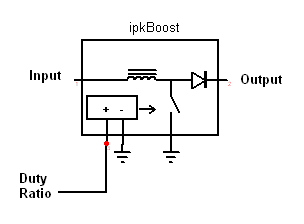Peak Current Control: The peak inductor current can be calculated based on the measured average value of the current, duty ratio and inductor voltage. Solving for the duty ratio results in the Middlbrook equation,  above.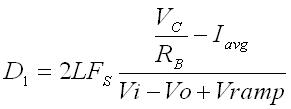(3) where      VC is the control input,      RB is the current sense resistor.      Vramp is the ramp compensation Inner Loop: A single injection loop cut was made using GTF theory, just after the Vavg signal. The resulting Bode plot exposes the high duty ratio instability of peak current control. Shown below is the phase margin vs. duty ratio resulting from the GFT analysis.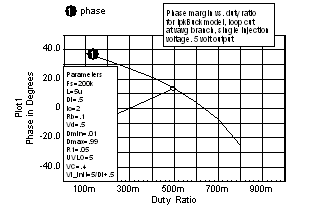Average Current Control: Solving for average current as a function of duty ratio yields: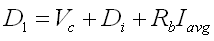(4) Di is the initial duty ratio and is used to remove offset, centering the output at the controlled value. That's the value used for the STATESET initial condition. The following average models that use STATESET are available from Intusoft for Buck, Boost and Buck/Boost(CCM):        peak current control        average current control        duty ratio control